# Normal text Arial 2. 3 7- The molecule of NH, is a) tetrahedral with bond angle...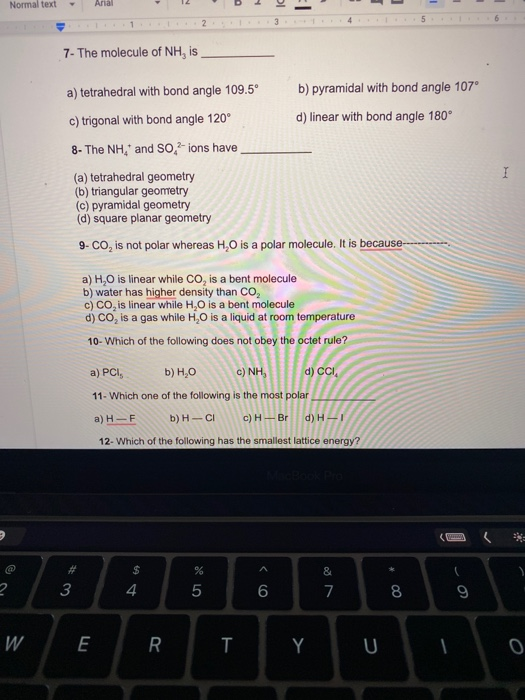Normal text Arial 2. 3 7- The molecule of NH, is a) tetrahedral with bond angle 109.5° b) pyramidal with bond angle 107° c) trigonal with bond angle 120° d) linear with bond angle 180° 8- The NH, and So, -ions have (a) tetrahedral geometry (b) triangular geometry c) pyramidal geometry (d) square planar geometry 9-CO, is not polar whereas H,0 is a polar molecule. It is because I a) H.O is linear while Co, is a bent molecule b) water has higher density than CO2 c) CO, is linear while H,O is a bent molecule d) Co, is a gas while HO is a liquid at room temperature 10- Which of the following does not obey the octet rule? a) PCI, b) H.O c) NHÀ d) CCI. 11- Which one of the following is the most polar a) H-F b) H-CI c) - Br d) H-1 12- Which of the following has the smallest lattice energy? \$ 4 % 5 3 & 7 6 8 9 W E R T Y U

1) NH3 is tetrahedral in shape with 107 degrees

hence option B is correct

2) NH4+ and SO42- both are tetrahedral in shape.

hence option A is correct

3) CO2 is linear in shape due to which both dipole moment are opposite to each other hence they cancelout each other.

hence option C is correct

4) In PCl5 phosphorus have 10 valence electrons hence it violated octet rule

hence option A is correct

5) fluorine is most ekectronegative element

hence option A is correct

#### Earn Coin

Coins can be redeemed for fabulous gifts.

Similar Homework Help Questions
• ### 7- The molecule of NH, is a) tetrahedral with bond angle 109.5° b) pyramidal with bond...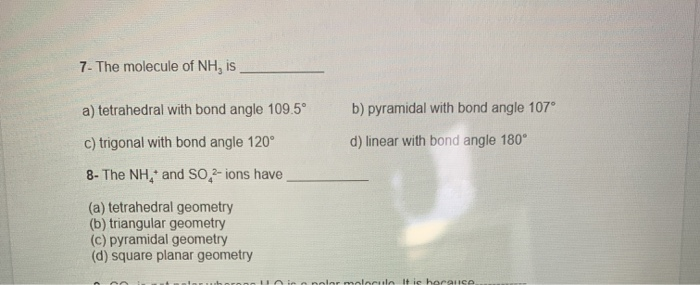7- The molecule of NH, is a) tetrahedral with bond angle 109.5° b) pyramidal with bond angle 107° d) linear with bond angle 180° C) trigonal with bond angle 120° 8- The NH,* and SO2-ions have (a) tetrahedral geometry (b) triangular geometry (c) pyramidal geometry (d) square planar geometry bolor molecule It is horance

• ### 2. Why do actual bond angles differ from the ideal bond angles? How does a lone...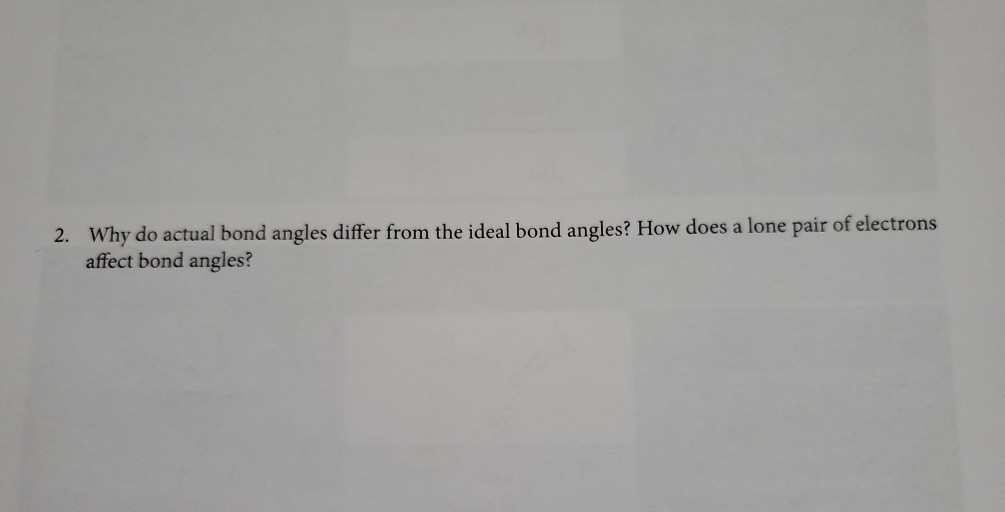2. Why do actual bond angles differ from the ideal bond angles? How does a lone pair of electrons affect bond angles? Lewis Dot structure Electronic geometry Molecular shape Ideal Bond angles Hybridization of central atom Does it contain polar bonds? Is the molecule polar? NH NH NH; tetrahedral Trigonal pyramidal 109 Spyes yes PES trigonal bipyramidal trigonal bipyramid Sp'd yes no and 90 Square Bris 90 and Octahedral Sn y es pyramidal yes 180 HCN HỮc : | linear...

• ### 180 No. of Molecule or Polyatomie lon Valence Electrons Lewis Structure Molecular Geometry Bond Angles Bond...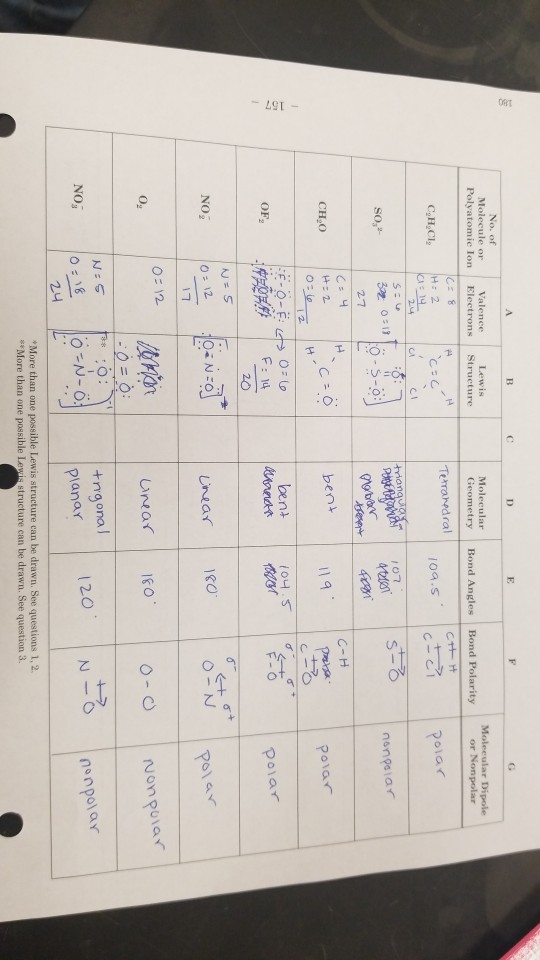180 No. of Molecule or Polyatomie lon Valence Electrons Lewis Structure Molecular Geometry Bond Angles Bond Polarity Molecular Dipole or Nonpolar Te amedral C.H.CI, H: 2 a = 14 109.5 lete polar S: triangular 107 S- SO, 410 nonpolar Botter Dobar 3270317 27 est (= 4 I ITO CHO Ic=0 bent 119 polar - 157 F-O-Ect 0= 6 bent 104.5 OF, F = 14 TOT polar 20 N=5 NO; Linear 180 polar |0=12 anion Unear 1800 Nonpolar 1 - 0...

• ### 10.) Specify the electron-domain geometry for the following two species using the VSEPR model: Xe...

10.) Specify the electron-domain geometry for the following two species using the VSEPR model: XeCl4 and IO4−. For each species, predict the bond angles based on the electron-domain geometry. (a)    XeCl4 geometry: linear trigonal planar    bent tetrahedral trigonal pyramidal trigonal bipyramidal seesaw T-shaped octahedral square pyramidal square planar pentagonal bipyramidal pentagonal pyramidal pentagonal planar bond angle: 60° 72°   90° 109.5° 120° 180° (b)    IO4− geometry: linear trigonal planar    bent tetrahedral trigonal pyramidal trigonal bipyramidal seesaw T-shaped octahedral square pyramidal square...

• ### are these answers correct? Academic Year 2019-20 Chem 06.100: Molecular Geometry and VSEPR Theory Species &...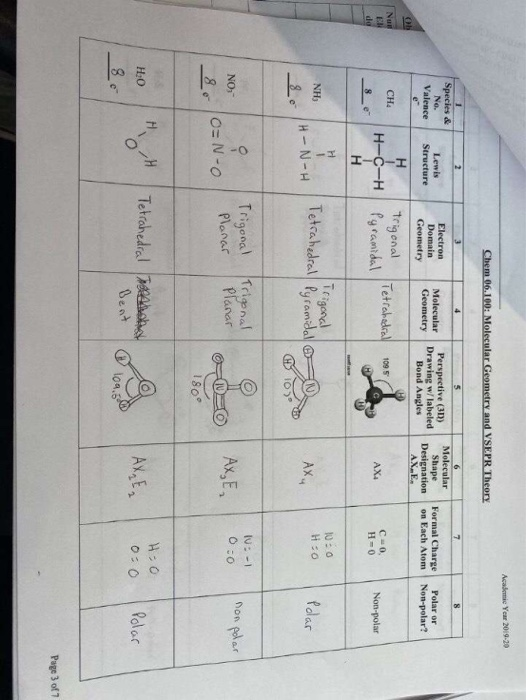are these answers correct? Academic Year 2019-20 Chem 06.100: Molecular Geometry and VSEPR Theory Species & No. Valence Lewis Structure Electron Domain Geometry Molecular Geometry Perspective (30) Drawing w/labeled Bond Angles Molecular Shape Designation AXE. Formal Charge on Each Atom Polar or Non-polar? н CHA Trigonal Pyramidal Tetrahedral 109.5 AX: H-C-H H CO H=0 Non-polar 8 NH) Trigonal Tetrahedral Pyramidal 100 HO TH-N-H Ах, Polar O Trigonal Planar NO, Trigonal Planar :-1 0=0 AX, E, I 1 non polar -...

• ### Specify the electron-domain geometry for the following two species using the VSEPR model: KrCl4 and BrO4....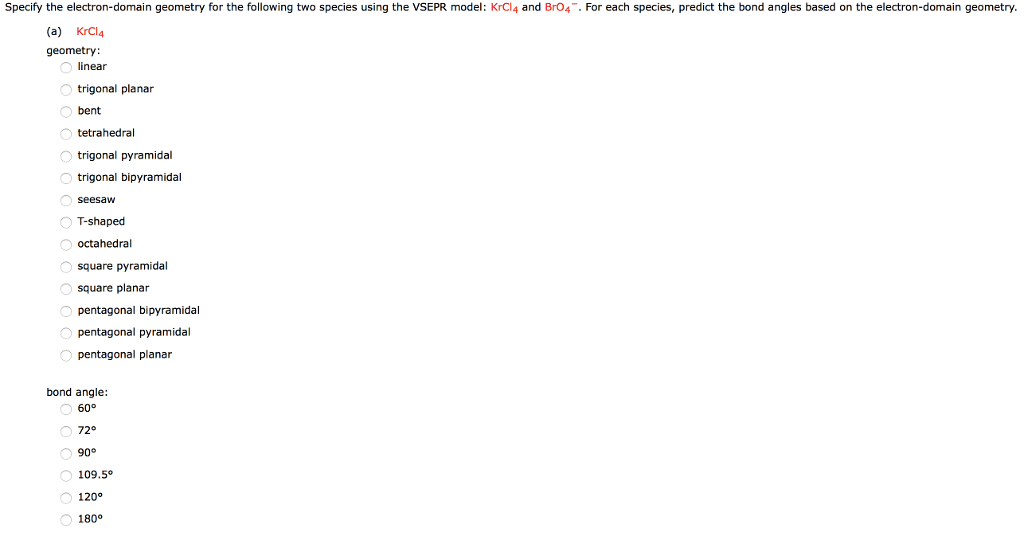Specify the electron-domain geometry for the following two species using the VSEPR model: KrCl4 and BrO4. For each species, predict the bond angles based on the electron-domain geometry. (a) KrCl4 geometry linear trigonal planar bent tetrahedral trigonal pyramidal trigonal bipyramidal T-shaped octahedral square pyramidal square planar pentagonal bipyramidal pentagonal pyramidal pentagonal planar bond angle: 72° 109.5° 120 180° (b) Br04 geometry: inear trigonal planar bent tetrahedral trigonal pyramidal trigonal bipyramidal seesaW T-shaped octahedral square pyramidal square planar pentagonal bipyramidal pentagonal...

• ### The electron geometries around G and G are a) best, trigonal planar b) tetrahedral, trigonal plaser...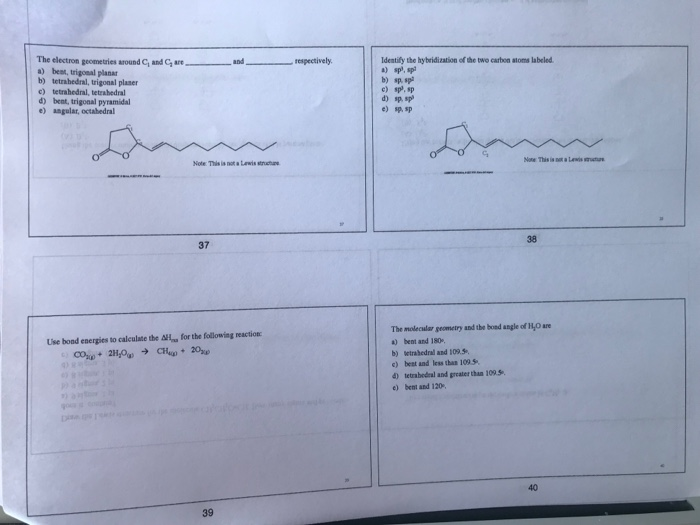The electron geometries around G and G are a) best, trigonal planar b) tetrahedral, trigonal plaser c) tetrahedral, tetrahedral d) bent, trigonal pyramidal e) angular, octahedral respectively. Identify the hybridization of the two carbon atoms labeled a) sp, sp and b) sp. sp c) sp, sp d) sp. sp cs Note This ia not a Lewis strucure 38 37 The molecular geometry and the boad angle of H,O are a) bent and 180, b) etrabedral and 109. s c) beet...

• ### Draw the Lewis structure of CIBr, showing all lone pairs. Identify the molecular geometry of CIBry...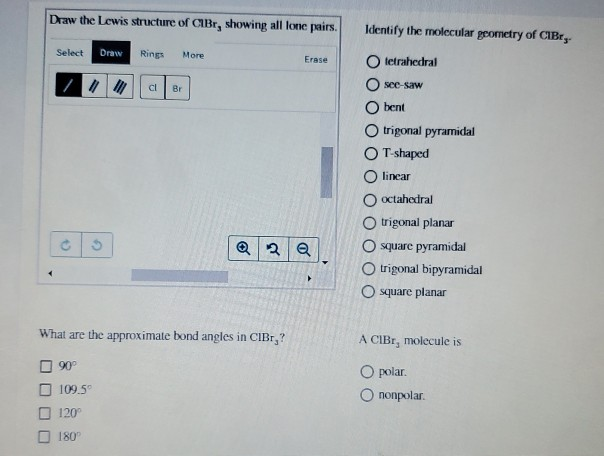Draw the Lewis structure of CIBr, showing all lone pairs. Identify the molecular geometry of CIBry Select Draw Rings More Erase tetrahedral see-saw bent trigonal pyramidal T-shaped O linear octahedral trigonal planar O square pyramidal trigonal bipyramidal square planar What are the approximate bond angles in CIBr,? A CIBr, molecule is O polar. Ononpolar. 90° 109.5 120 180

• ### Draw the Lewis structure of XeCl. Include all lone pairs. Identify the molecular geometry of Xeci,...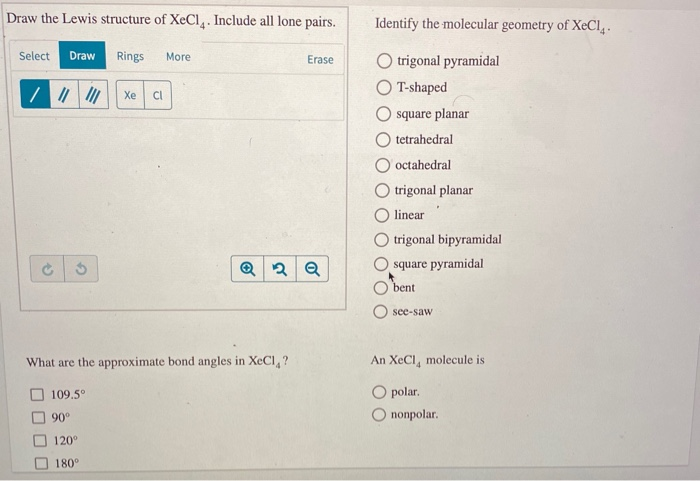Draw the Lewis structure of XeCl. Include all lone pairs. Identify the molecular geometry of Xeci, Select Draw Rings More Erase trigonal pyramidal T-shaped square planar O tetrahedral O octahedral trigonal planar linear trigonal bipyramidal square pyramidal bent O see-saw What are the approximate bond angles in XeCl,? An XeCl, molecule is O polar O nonpolar. 109.5° 90° 120° 180°

• ### Localized Electron Bond Model -- Structure and Bonding (4 parts -- 3 molecules) The XeO3F2 molecu...

Localized Electron Bond Model -- Structure and Bonding (4 parts -- 3 molecules) The XeO3F2 molecule can be said to use which type of Xe hybrid orbitals for sigma-bonding? sp sp2 sp3 dsp3 d2sp3 VSEPR theory predicts which arrangement of bonds and lone pairs of electrons about the central Xe atom in XeO3F2? linear trigonal planar tetrahedral trigonal bipyramidal octahedral Is the XeO3F2 molecule polar or non-polar? polar non-polar 1pts Tries 0/5 The XeO3F2 molecule displays which bond angles (in...